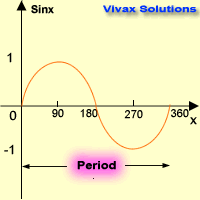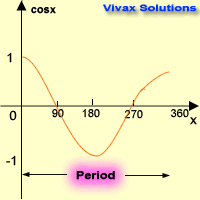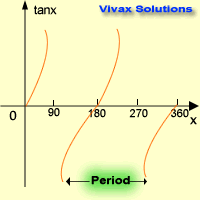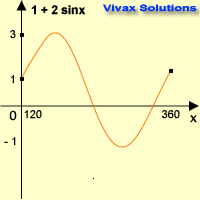# Mathematics

### Trigonometric Curves

The three main trigonometric graphs are:

• Sine graph
• Cosine graph
• Tan graph

#### Sine Graphx0 sin x 0 0 90 1 180 0 270 -1 360 0

Characteristics:

• Period is 3600. It repeats itself every 360 degrees.
• Maximum value = +1
• Minimum value = -1

#### Cosine Graphx0 cos x 0 1 90 0 180 -1 270 0 360 1

Characteristics:

• Period is 3600. It repeats itself every 360 degrees.
• Maximum value = +1
• Minimum value = -1

#### Tan Graphx0 tan x 0 0 90 ∞ 180 0 270 ∞ 360 0

Characteristics:

• Period is 1800. It repeats itself every 180 degrees.
• Maximum value = +∞
• Minimum value = -∞

#### Practice for Trigonometric Curves - interactive

You may move the grid so that you can see the behaviour of the curves for the bigger values of the angles - on both sides.

#### Transformation of a Graphx0 sin x 2 sin x 1 + 2 sin x 0 0 0 1 90 1 2 3 180 0 0 1 270 -1 -2 -1 360 0 0 1

This is stretched by a factor 2 parallel to the y-axis and then moved up by 1 - a translation of 1 in the positive y-axis.

Now, in order to complement what you have just learnt, work out the following questions:

>
1. Sketch the following graphs:
• 2 cos x
• 1 - 3 sin x
• 2 tan x
• 2 + 3 sin x
2. Find the period, maximum value and minimum value of the following functions:
• 3 cos x
• 1 - 2sin 2x
• 3 cos 2x
• 2 + 3 sin x/2Maths is challenging; so is finding the right book. K A Stroud, in this book, cleverly managed to make all the major topics crystal clear with plenty of examples; popularity of the book speak for itself - 7th edition in print.

### Recommended - GCSE & iGCSEThis is the best book available for the new GCSE(9-1) specification and iGCSE: there are plenty of worked examples; a really good collection of problems for practising; every single topic is adequately covered; the topics are organized in a logical order.

### Recommended for A LevelThis is the best book that can be recommended for the new A Level - Edexcel board: it covers every single topic in detail;lots of worked examples; ample problems for practising; beautifully and clearly presented.